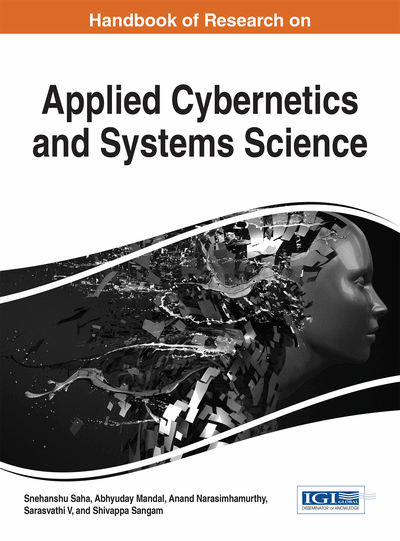# Application of Corona Product of Graphs in Computing Topological Indices of Some Special Chemical Graphs

Nilanjan De (Calcutta Institute of Engineering and Management, India)
DOI: 10.4018/978-1-5225-2498-4.ch004
Available
\$33.75
List Price: \$37.50
10% Discount:-\$3.75
TOTAL SAVINGS: \$3.75

## Abstract

Graph operations play a very important role in mathematical chemistry, since some chemically interesting graphs can be obtained from some simpler graphs by different graph operations. In this chapter, some eccentricity based topological indices such as the total eccentricity index, eccentric connectivity index, modified eccentric connectivity index and connective eccentricity index and their respective polynomial versions of corona product of two graphs have been studied and also these indices of some important classes of chemically interesting molecular graphs are determined by specializing the components of corona product of graphs.
Chapter Preview
Top

## Background

Different topological indices have been defined and many of them have found applications as means to model chemical, pharmaceutical and other properties of molecules. Thus topological indices are convenient means of translating chemical constitution into numerical values. There are various types of topological indices; among these in this chapter we consider mainly vertex degree cum eccentricity based topological indices. The total eccentricity index of a graph is defined as

.

Fathalikhani et al. (2014) have studied total eccentricity of some graph operations. Some studies on total eccentricity index are also found in literature (Dankelmann, Goddard & Swart, 2004; De, Nayeem & Pal, 2014, 2015).The eccentric connectivity index of a graph G was proposed by Sharma, Goswami and Madan (1997) and is defined as

.

## Complete Chapter List

Search this Book:
Reset